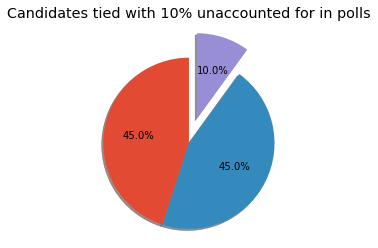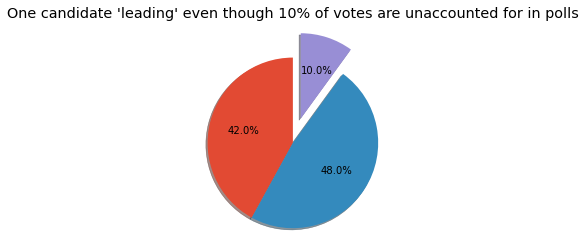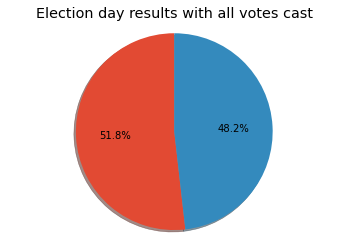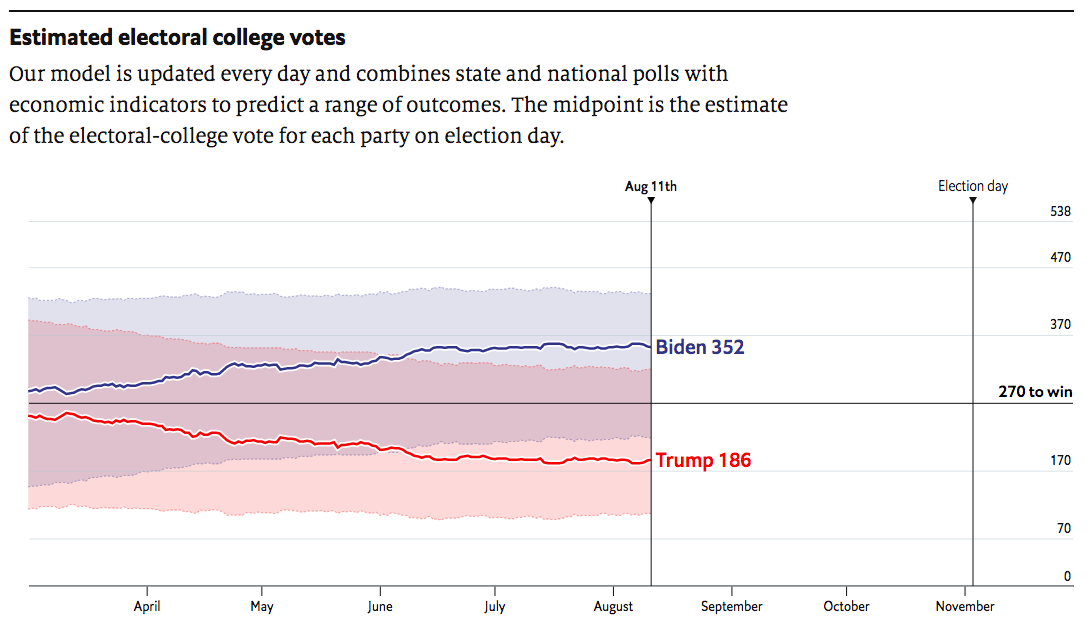# How Close is the 2020 U.S. Presidential Election Race?¶

We see the latest poll updates and understand polling averages, but 2016 jaded many of us in terms of trusting poll numbers. We can use weighted poll averages based upon the quality of the poll conducted and monte carlo simulations to provide candidate probabilities for each state.In :
%matplotlib inline
import numpy as np, pandas as pd, plotly.graph_objects as go
import random
import datetime
from collections import Counter
import warnings
import matplotlib.pyplot as plt
random.seed(2020)
warnings.filterwarnings('ignore')
plt.style.use('ggplot')


### Polls Only Probabilities¶

If we look at all the states where a candidate has at least a 50% probability without any undecided voters, we get our first benchmark for the state of the race.

In :
df = pd.read_csv('https://raw.githubusercontent.com/ahoaglandnu/election2020/master/results/results_20200811.csv')
print('Biden electoral college votes with at least a 50% probability:', np.sum(df['ec'][df['Biden_probability'] >= .5]))
print()
print('Trump electoral college votes with at least a 50% probability:', np.sum(df['ec'][df['Trump_probability'] >= .5]))

Biden electoral college votes with at least a 50% probability: 262

Trump electoral college votes with at least a 50% probability: 111


You may have noticed that the total does not add up to 538, the total votes in the Electoral College. That is because we are only looking at states where one candidate has at least a 50% majority.

### But we have to consider what happened in 2016¶

Undecided voters swayed election results in states where one candidate did not have a 50% or higher majority. When we add ALL undecided votes to Trump, we get new probabilities for each state.

In :
print('Biden electoral college votes if ALL undecided voters go for Trump:',np.sum(df['ec'][df['biden_alt_prob'] >= .5]))
print()
print('Trump electoral college votes if ALL undecided voters go for Trump:',np.sum(df['ec'][df['trump_alt_prob'] >= .5]))

Biden electoral college votes if ALL undecided voters go for Trump: 258

Trump electoral college votes if ALL undecided voters go for Trump: 280


Polls and simple corrections to polls give us two different outcomes. This is our first clear indication that the race is far closer and more complex than it may initially appear.

# How the undecided vote can determine the winner¶

On election day, the votes for each candidate will add up to 100%. When polls do not have a candidate with a majority of the votes, then the candidate "winning" the race is not necessarily in the lead.

We can illustrate this scenario by examining a simple pie chart.

In :
sizes = [45, 45, 10]
explode = (0, 0, 0.3)
fig1, ax1 = plt.subplots()
ax1.pie(sizes, explode=explode, autopct='%1.1f%%',
ax1.axis('equal')
plt.title("Candidates tied with 10% unaccounted for in polls")
plt.show()In :
sizes = [42, 48, 10]
explode = (0, 0, 0.3)
fig1, ax1 = plt.subplots()
ax1.pie(sizes, explode=explode, autopct='%1.1f%%',
ax1.axis('equal')
plt.title("One candidate 'leading' even though 10% of votes are unaccounted for in polls")
plt.show()In :
sizes = [51.8, 48.2]
fig1, ax1 = plt.subplots()
ax1.pie(sizes, autopct='%1.1f%%',
ax1.axis('equal')
plt.title('Election day results with all votes cast')
plt.show()On election day, the majority of undecided voters in polling voted for one candidate.

This is the outcome we saw in 2016 in several states that ultimately determined the winner of the election.

# Randomly Distribute the Undecided Vote¶

The most mathematically unbiased method is to randomly distribute undecided voters to each candidate.

A random number generator will pick a value between .01 and .99.

One candidate will receive that percentage of the undecided votes for the state; the other candidate the remaining undecided votes.

For the polling results, we will use normal distribution to randomly choose the number of votes cast for each candidate using the weighted polling as the average assuming a 3 percent margin of error.

The undecided votes will then be added to the the polling results and the candidate with the highest number of votes will win that state.

We will do the above process for each state 20,000 times. The number of times a candidate wins each state divided by the number of simulations (20,000) will be our probability the candidate will win the state.

In :
def moe(x, y, n):
min_x = x - n
max_x = x + n
std_x = (max_x - min_x) / 4
min_y = y - n
max_y = y + n
std_y = (max_y - min_y) / 4
return round(random.gauss(x, std_x),1), round(random.gauss(y, std_y),1)
def distro_sim(x, y, n=3, num_sims=20000):
u = 100 - (x + y)
x_wins = 0
y_wins = 0
for i in range(num_sims):
rand = np.random.uniform(low=0.01,high=.99)
x1 = x + (u * rand)
y1 = y + (u * (1 - rand))
x1, y1 = moe(x1, y1, n)
if x1 > y1:
x_wins += 1
else:
y_wins += 1
return x_wins/num_sims, y_wins/num_sims

In :
b = df['Biden_avg'].values
t = df['Trump_avg'].values
rand_prob = []
for x, y in zip(b,t):
rp = distro_sim(x,y)
rand_prob.append(rp)
df['biden_rand_prob'] = [i for i in rand_prob]
df['trump_rand_prob'] = [i for i in rand_prob]
df.loc[(df['cook'] == 'Solid Dem') & (df['Biden_avg'].isnull()),'biden_rand_prob'] = 1.0
df.loc[(df['cook'] == 'Solid Dem') & (df['Biden_avg'].isnull()),'trump_rand_prob'] = 0.0
df.loc[(df['cook'] == 'Solid Rep') & (df['Trump_avg'].isnull()),'biden_rand_prob'] = 0.0
df.loc[(df['cook'] == 'Solid Rep') & (df['Trump_avg'].isnull()),'trump_rand_prob'] = 1.0
print('Biden electoral college votes with randomly distributed undecided votes:', np.sum(df['ec'][df['biden_rand_prob'] >= .5]))
print()

Biden electoral college votes with randomly distributed undecided votes: 352



### That looks like a landslide. Is anyone else getting that result?¶

Yes. At the time of creation, The Economist had the same forecast### "But we have to consider what happened in 2016"¶

I hear this all the time and I agree.

Even if forecast models are constructed in a manner that worked for other elections in the past, there is a prevailing sense that the 2016 polling errors can or will occur again in 2020.

To answer this concern, we will identify the 2020 battleground states and recreate the 2016 polling error.

# Battleground States According to the Cook Political Report¶

States labeled as toss up and leaning will be considered battleground states.

Note the poll-based probabilities below. We see divergences between the probabilities and qualitative assessments.

In :
df[df['cook'].str.startswith('Lean R', na=False)][['State','cook','biden_rand_prob','trump_rand_prob','Biden_avg','Trump_avg']]

Out:
State cook biden_rand_prob trump_rand_prob Biden_avg Trump_avg
11 iowa Lean Rep 0.41350 0.58650 44.5 46.0
27 ohio Lean Rep 0.77495 0.22505 48.4 44.4
31 texas Lean Rep 0.27890 0.72110 43.7 47.5
In :
df[df['cook'].str.startswith('Lean D', na=False)][['State','cook','biden_rand_prob','trump_rand_prob','Biden_avg','Trump_avg']]

Out:
State cook biden_rand_prob trump_rand_prob Biden_avg Trump_avg
16 michigan Lean Dem 0.97465 0.02535 50.4 40.4
17 minnesota Lean Dem 0.89155 0.10845 49.3 43.1
22 new hampshire Lean Dem 0.96130 0.03870 50.3 42.7
28 pennsylvania Lean Dem 0.79520 0.20480 48.5 44.0
35 wisconsin Lean Dem 0.86990 0.13010 48.8 42.0
In :
df[df['cook'].str.startswith('Toss', na=False)][['State','cook','biden_rand_prob','trump_rand_prob','Biden_avg','Trump_avg']]

Out:
State cook biden_rand_prob trump_rand_prob Biden_avg Trump_avg
2 arizona Toss Up 0.54735 0.45265 46.4 45.6
8 florida Toss Up 0.95095 0.04905 50.7 46.0
9 georgia Toss Up 0.21555 0.78445 44.6 48.5
26 north carolina Toss Up 0.66760 0.33240 47.7 45.3

### Factoring in what we witnessed for the 2016 election¶

States that were Lean Dem and Toss Up in 2016 had undecided voters overwhelming vote for Trump.

To replicate this, we will use our all undecided votes for Trump probabilities for these states unless Biden is polling over 50% in a Lean Dem state.

In :
def qual_correction (row):
if row['cook'] == "Lean Dem" and row['Biden_avg'] < 50:
return row['trump_alt_prob']
if row['cook'] == "Lean Rep" :
return row['trump_alt_prob']
if row['cook'] == 'Toss Up' :
return row['trump_alt_prob']
else:
return row['trump_rand_prob']
def qual_correction_opp (row):
if row['cook'] == "Lean Dem" and row['Biden_avg'] < 50:
return row['biden_alt_prob']
if row['cook'] == "Lean Rep" :
return row['biden_alt_prob']
if row['cook'] == 'Toss Up' :
return row['biden_alt_prob']
else:
return row['biden_rand_prob']

In :
df['biden_qual_prob'] = df['biden_rand_prob']
df['trump_qual_prob'] = df['trump_rand_prob']
df['trump_qual_prob'] = df.apply (lambda row: qual_correction(row), axis=1)
df['biden_qual_prob'] = df.apply (lambda row: qual_correction_opp(row), axis=1)


#### The adjusted poll-based probabilities align with some qualitative assessments while making other states more competitive.¶

In :
df[df['cook'].str.startswith('Lean R', na=False)][['State','cook','biden_qual_prob','trump_qual_prob','Biden_avg','Trump_avg']]

Out:
State cook biden_qual_prob trump_qual_prob Biden_avg Trump_avg
11 iowa Lean Rep 0.00000 1.00000 44.5 46.0
27 ohio Lean Rep 0.04185 0.95815 48.4 44.4
31 texas Lean Rep 0.00000 1.00000 43.7 47.5
In :
df[df['cook'].str.startswith('Lean D', na=False)][['State','cook','biden_qual_prob','trump_qual_prob','Biden_avg','Trump_avg']]

Out:
State cook biden_qual_prob trump_qual_prob Biden_avg Trump_avg
16 michigan Lean Dem 0.97465 0.02535 50.4 40.4
17 minnesota Lean Dem 0.17085 0.82915 49.3 43.1
22 new hampshire Lean Dem 0.96130 0.03870 50.3 42.7
28 pennsylvania Lean Dem 0.05240 0.94760 48.5 44.0
35 wisconsin Lean Dem 0.08605 0.91395 48.8 42.0
In :
df[df['cook'].str.startswith('Toss', na=False)][['State','cook','biden_qual_prob','trump_qual_prob','Biden_avg','Trump_avg']]

Out:
State cook biden_qual_prob trump_qual_prob Biden_avg Trump_avg
2 arizona Toss Up 0.00015 0.99985 46.4 45.6
8 florida Toss Up 0.59325 0.40675 50.7 46.0
9 georgia Toss Up 0.00000 1.00000 44.6 48.5
26 north carolina Toss Up 0.00815 0.99185 47.7 45.3

### If all undecided voters go for Trump in battleground states¶

In :
df2 = df[['State','code','ec','cook','Biden_avg','Trump_avg','biden_qual_prob','trump_qual_prob']]
for col in df2.columns:
df2[col] = df2[col].astype(str)
df2['text'] = df2['State'] + '<br>' + \
'Electoral College Votes '+ df2['ec'] +  '<br>' + \
'Cook Political Report: ' + df2['cook'] + '<br>' + \
'Biden ' + df2['Biden_avg'] + ' Trump ' + df2['Trump_avg'] + '<br>'\
'Biden probability ' + df2['biden_qual_prob'] + '<br>'\
'Trump probability ' + df2['trump_qual_prob']

In :
fig = go.Figure(data=go.Choropleth(
locations=df2['code'],
z=df2['biden_qual_prob'].astype(float),
locationmode='USA-states',
colorscale='RdBu',
autocolorscale=False,
text=df2['text'],
marker_line_color='white',
colorbar_title="Biden Probability"
))
fig.update_layout(
title_text='State probabilities with battleground undecided voters favoring Trump',
geo = dict(
scope='usa',
projection=go.layout.geo.Projection(type = 'albers usa'),
showlakes=False),
)

fig.show()